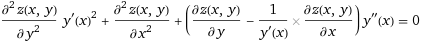# Looking for help with this PDE

• I
• chacal10

#### chacal10

TL;DR Summary
I have not been able to solve this PDE; need some help plsas you can see, z(x,y) is a function of x, y; and y is a function of x, therefore y'(x) is the total derivative of "y" respect to "x", and y"(x) is the 2nd derivative. y'(x)^2 is just the square of the derivative of y respect to x

I don't have boundary or initial conditions, so you can make up any if that simplifies finding the solution

Thank you !

Have you tried the separation of variables strategy?

Assume ##Z(x,y) = X(x)Y(y)##

Let me try that. I think the problem with separation of variables is the total derivative terms y'(x) and y"(x) that multiply the partial derivatives

I don't know much about PDEs but I see you're looking for two functions here, shouldn't there be a system of coupled equations instead of just one?

@TheDS1337
Is actually one function Z(x,y), but "y" itself is a function of "x" (unknown at this time)
What I am trying to find as the "solution" to this PDE is Z(x,y), i.e. something like "...Z(x,y) = A*cos(y-wx) + exp(-y*x)..." or something like that.
Note that the first 2 parts of the PDE look suspiciously close to a wave equation (if you replace "x" by "t"), but then the 3rd part messes the whole thing up

Thank you all!